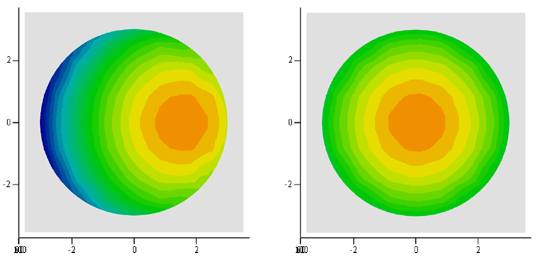Case 11: Convex Lens in Dome Coater

In Case 10, we studied the thickness distribution on a spherical convex lens across a diameter in the direction of the fixture radius. Equally important may be the thickness distribution in the perpendicular direction. For this we may compute for points in a diameter along the Y direction, or we may compute for points in a 2D grid to obtain thickness contours. In this example we carry out the latter.

​      We import the 3D lens surface file that we used in Case 5. To study the thickness distribution on the lens that is the 4th from the planet center in the last case, we move the surface to that location. We then compute for two lens orientations: 1) the lens is positioned tangentially to the dome, and 2) the lens is pivoted 11.5 degrees from the tangential orientation, as shown in the surface views below. The thickness contours with and without pivoting are also shown below: the left column is before pivoting and right column is after pivoting.

The pivoting clearly corrected the severe asymmetry of the thickness. The overall peak-to-valley nonuniformity before and after pivoting is 13.6% and 6.6%, respectively.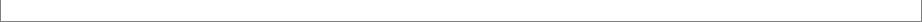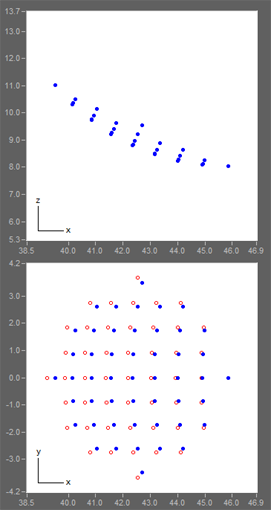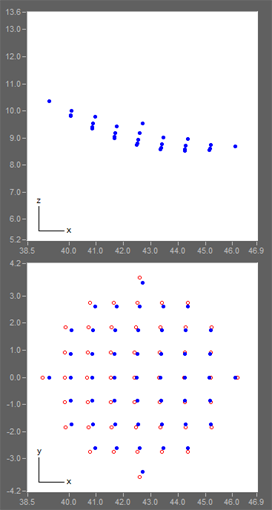Tangential to dome
maximum=99.66; minimum=75.74
Pivoted from dome
maximum=99.75; minimum=87.33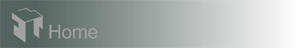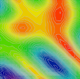Hardware requirements
Request a Free Trial